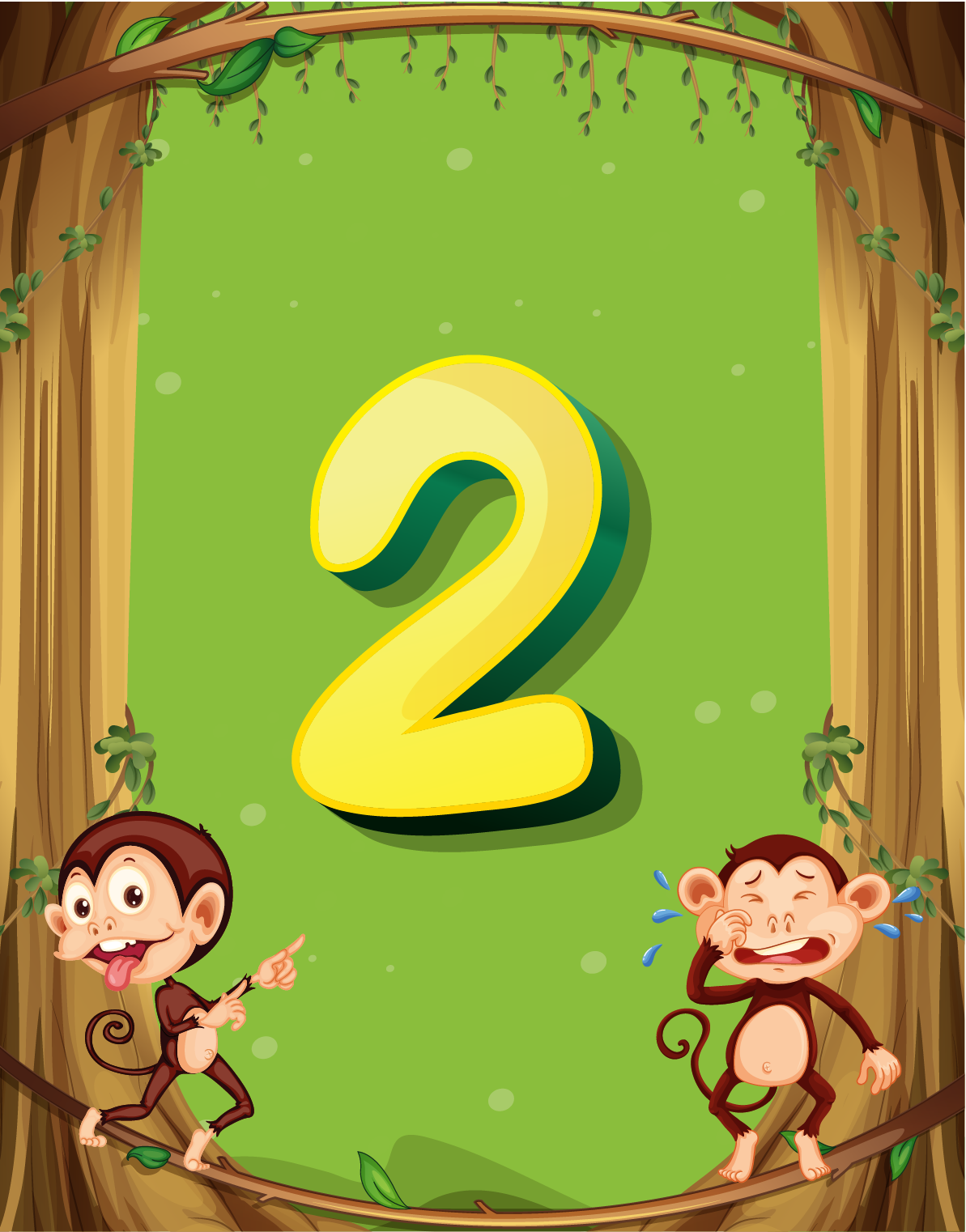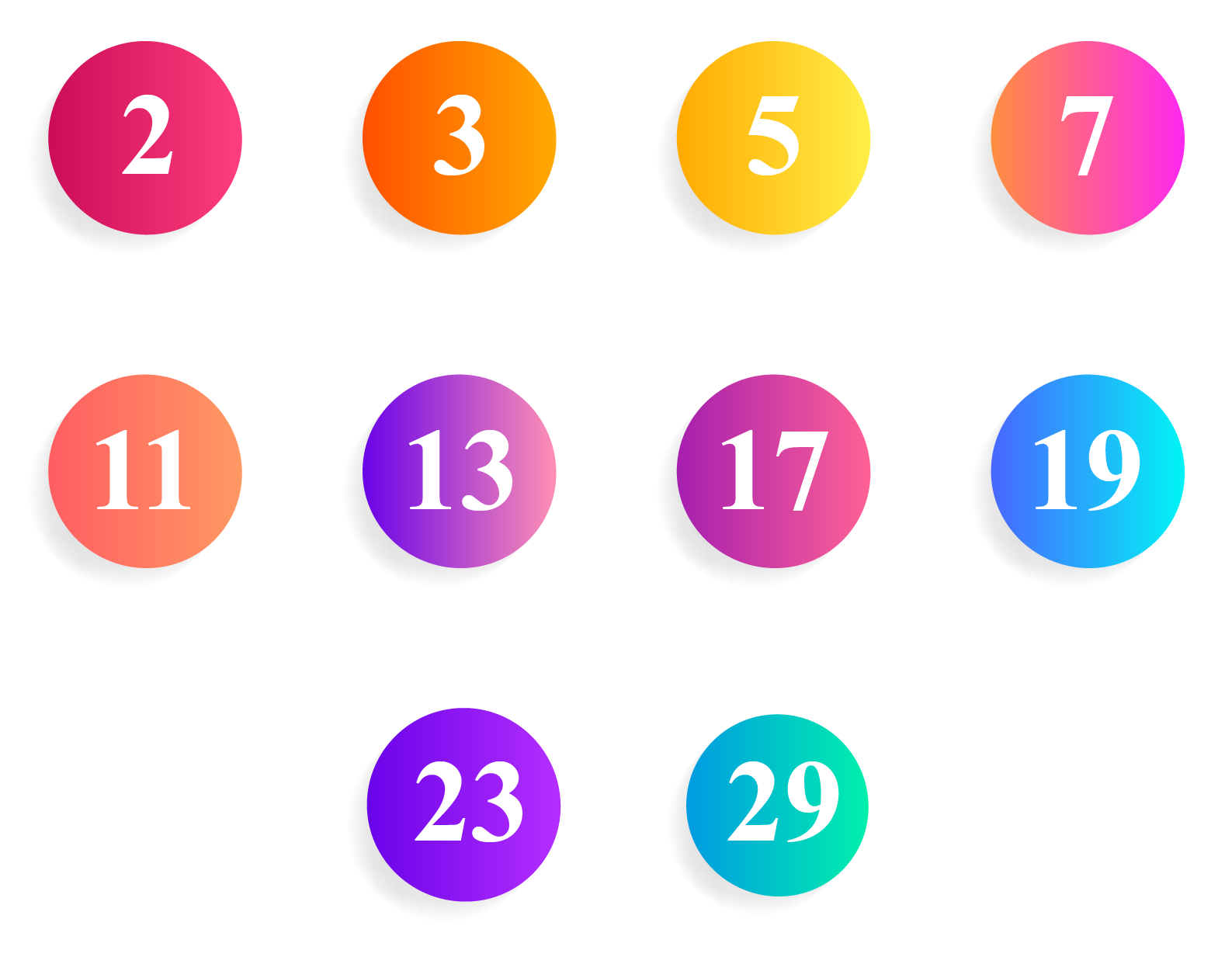# Is 2 a Prime Number

Is 2 a Prime Number
Go back to  'Prime Numbers'

It is believed that good things come in pairs.

The number 2 represents 2 units or a pair.The binary number system, also known as a base-2 system, is the foundation of all the coding and technology.

We know that the human body has a great connection with the number '2'. We have 2 eyes, 2 ears, 2 hands, and so on.

Now, let's learn some math behind this number. Let's find out if 2 a prime number.## What Is a Prime Number?

### Definition

A prime number is a natural number that has no factors other than 1 and itself.

In other words, it is divisible only by 1 and itself.

Example: 2,3,5,7,11 and so on.

## Is 2 a Prime Number?

Let us look at the factors of 2

The factors of 2 are 1 and 2, that is, no other factors other than 1 and itself.

So, 2 is a prime number.

Considering the above explanation, is 3 a prime number?

Let's find out!

The factors of 3 are 1 and 3, that is, no other factors other than 1 and itself.

So, 3 is a prime number.

## Is 2 a Composite Number?

2 is not a composite number as it cannot be written as a product of two natural numbers other than 1 and itself.

2 is the only even prime number.

All even numbers are composite, except 2

## Solved Examples

 Example 1

Kate says "All odd numbers are prime numbers."

Do you agree with her? Explain with a suitable example.

Solution

All odd numbers are not prime numbers.

Let us take the example of 9.

Even though 9 is an odd number, it has 1, 3, and 9 as its factors.

So, it is a composite number.

A few other examples of odd composite numbers are 15, 21, 27, and so on.

 Kate's statement is not true.
 Example 2

List the first ten prime numbers.

Solution

Using divisibility rules, we can list the first ten prime numbers as:2, 3, 5, 7, 11, 13, 17, 19, 23, 29Challenging Question
1. Find three different prime numbers which when added together give the sum as 40

## Interactive Questions

Here are a few activities for you to practice. Select/Type your answer and click the "Check Answer" button to see the result.

## Let's Summarize

The mini-lesson targeted the fascinating concept of 'is 2 a prime number'. The math journey around 'is 2 a prime number' starts with what a student already knows, and goes on to creatively crafting a fresh concept in the young minds. Done in a way that not only it is relatable and easy to grasp, but also will stay with them forever. Here lies the magic with Cuemath.

At Cuemath, our team of math experts is dedicated to making learning fun for our favorite readers, the students!

Through an interactive and engaging learning-teaching-learning approach, the teachers explore all angles of a topic.

Be it worksheets, online classes, doubt sessions, or any other form of relation, it’s the logical thinking and smart learning approach that we, at Cuemath, believe in.

## 1. What are co-primes?

Two numbers are said to be co-prime if they do not have any common factors except 1

For example: (2,5), (2,9), (9,16) are co-primes.

The factors of 16 can be listed as 1, 2, 4, 8, 16, and the factors of 9 are 1, 3, 9

16 and 9 do not have any common factors except 1. So, they are co-primes.

## 2. Are 2 and 3 twin primes?

No, 2 and 3 are not twin primes.

Pairs of primes that differ by two and have a composite number in between them are called twin primes.

For example: (3,5), (5,7), (11,13), and so on.

## 3. What is 1 called if it is not prime?

1 is neither prime nor composite.

It is the smallest natural number.

More Important Topics
Numbers
Algebra
Geometry
Measurement
Money
Data
Trigonometry
Calculus